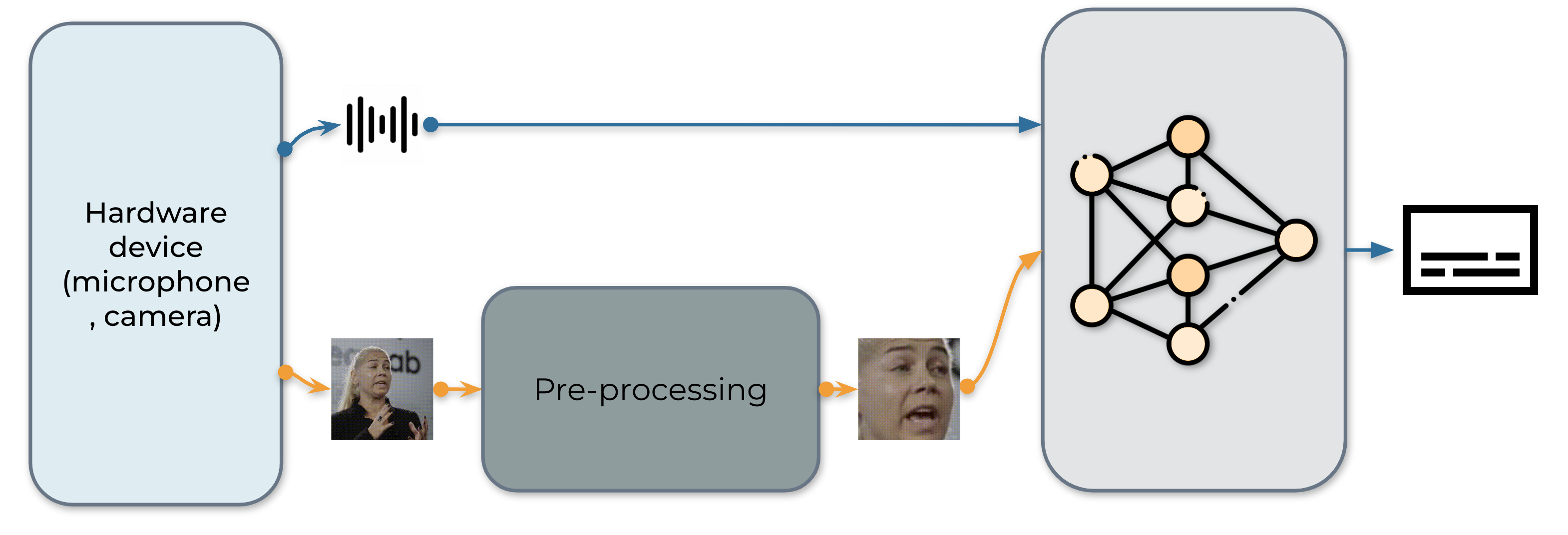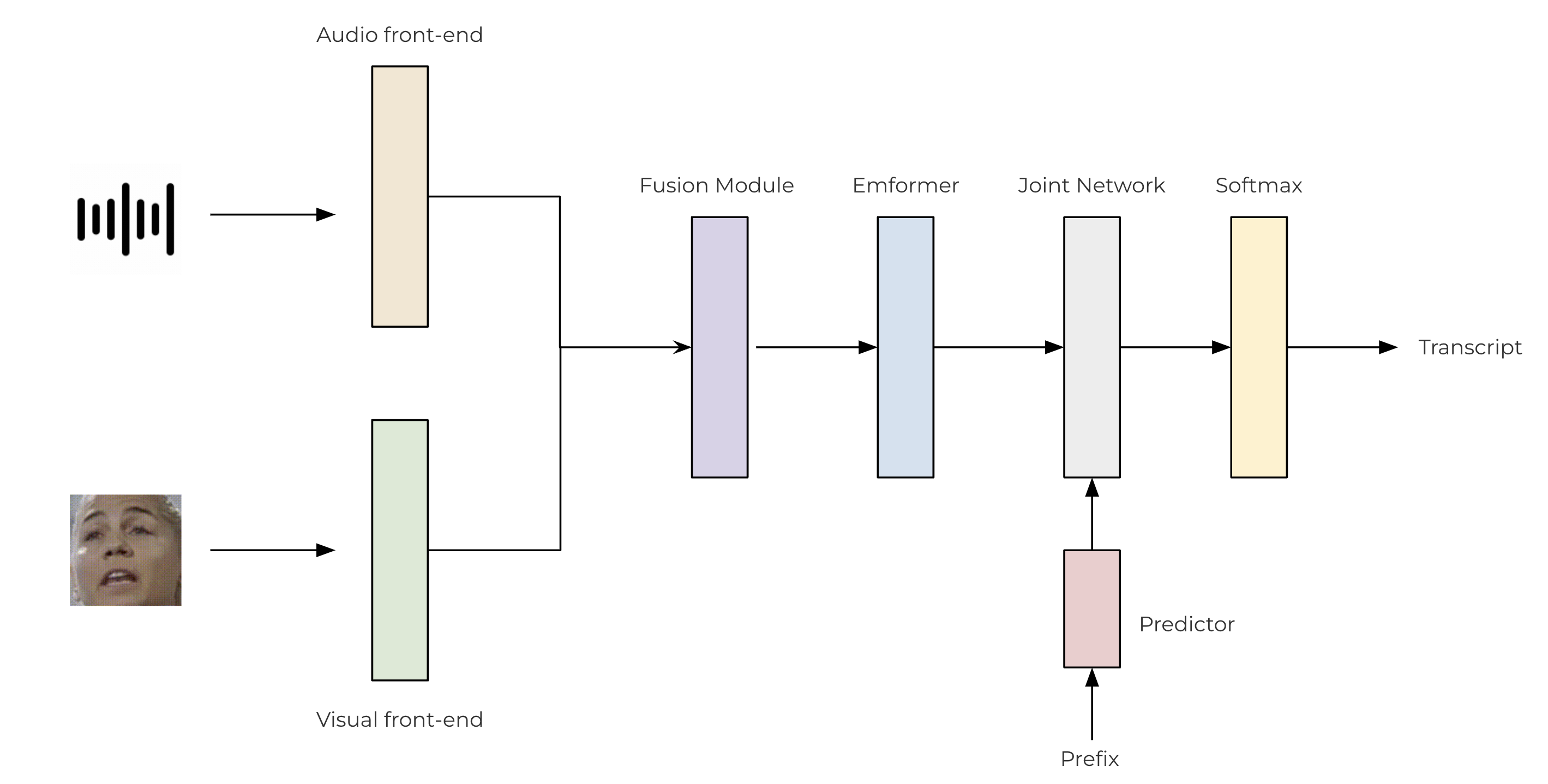Shortcuts

# Device AV-ASR with Emformer RNN-T¶

Author: Pingchuan Ma, Moto Hira.

This tutorial shows how to run on-device audio-visual speech recognition (AV-ASR, or AVSR) with TorchAudio on a streaming device input, i.e. microphone on laptop. AV-ASR is the task of transcribing text from audio and visual streams, which has recently attracted a lot of research attention due to its robustness against noise.

Note

This tutorial requires ffmpeg, sentencepiece, mediapipe, opencv-python and scikit-image libraries.

There are multiple ways to install ffmpeg libraries. If you are using Anaconda Python distribution, conda install -c conda-forge 'ffmpeg<7' will install compatible FFmpeg libraries.

You can run pip install sentencepiece mediapipe opencv-python scikit-image to install the other libraries mentioned.

Note

To run this tutorial, please make sure you are in the tutorial folder.

Note

We tested the tutorial on torchaudio version 2.0.2 on Macbook Pro (M1 Pro).

import numpy as np
import sentencepiece as spm
import torch
import torchaudio
import torchvision


## Overview¶

The real-time AV-ASR system is presented as follows, which consists of three components, a data collection module, a pre-processing module and an end-to-end model. The data collection module is hardware, such as a microphone and camera. Its role is to collect information from the real world. Once the information is collected, the pre-processing module location and crop out face. Next, we feed the raw audio stream and the pre-processed video stream into our end-to-end model for inference.## 1. Data acquisition¶

Firstly, we define the function to collect videos from microphone and camera. To be specific, we use StreamReader class for the purpose of data collection, which supports capturing audio/video from microphone and camera. For the detailed usage of this class, please refer to the tutorial.

def stream(q, format, option, src, segment_length, sample_rate):

print(streamer.get_src_stream_info(0))
print(streamer.get_src_stream_info(1))
print("Streaming...")
print()
for (chunk_v, chunk_a) in streamer.stream(timeout=-1, backoff=1.0):
q.put([chunk_v, chunk_a])

class ContextCacher:
def __init__(self, segment_length: int, context_length: int, rate_ratio: int):
self.segment_length = segment_length
self.context_length = context_length

self.context_length_v = context_length
self.context_length_a = context_length * rate_ratio
self.context_v = torch.zeros([self.context_length_v, 3, 340, 600])
self.context_a = torch.zeros([self.context_length_a, 1])

def __call__(self, chunk_v, chunk_a):
if chunk_v.size(0) < self.segment_length:
chunk_v = torch.nn.functional.pad(chunk_v, (0, 0, 0, 0, 0, 0, 0, self.segment_length - chunk_v.size(0)))
if chunk_a.size(0) < self.segment_length * 640:
chunk_a = torch.nn.functional.pad(chunk_a, (0, 0, 0, self.segment_length * 640 - chunk_a.size(0)))

if self.context_length == 0:
return chunk_v.float(), chunk_a.float()
else:
chunk_with_context_v = torch.cat((self.context_v, chunk_v))
chunk_with_context_a = torch.cat((self.context_a, chunk_a))
self.context_v = chunk_v[-self.context_length_v :]
self.context_a = chunk_a[-self.context_length_a :]
return chunk_with_context_v.float(), chunk_with_context_a.float()


## 2. Pre-processing¶

Before feeding the raw stream into our model, each video sequence has to undergo a specific pre-processing procedure. This involves three critical steps. The first step is to perform face detection. Following that, each individual frame is aligned to a referenced frame, commonly known as the mean face, in order to normalize rotation and size differences across frames. The final step in the pre-processing module is to crop the face region from the aligned face image.Original Detected Transformed Cropped
import sys

sys.path.insert(0, "../../examples")

from avsr.data_prep.detectors.mediapipe.detector import LandmarksDetector
from avsr.data_prep.detectors.mediapipe.video_process import VideoProcess

class FunctionalModule(torch.nn.Module):
def __init__(self, functional):
super().__init__()
self.functional = functional

def forward(self, input):
return self.functional(input)

class Preprocessing(torch.nn.Module):
def __init__(self):
super().__init__()
self.landmarks_detector = LandmarksDetector()
self.video_process = VideoProcess()
self.video_transform = torch.nn.Sequential(
FunctionalModule(
lambda n: [(lambda x: torchvision.transforms.functional.resize(x, 44, antialias=True))(i) for i in n]
),
FunctionalModule(lambda x: torch.stack(x)),
torchvision.transforms.Normalize(0.0, 255.0),
torchvision.transforms.Grayscale(),
torchvision.transforms.Normalize(0.421, 0.165),
)

def forward(self, audio, video):
video = video.permute(0, 2, 3, 1).cpu().numpy().astype(np.uint8)
landmarks = self.landmarks_detector(video)
video = self.video_process(video, landmarks)
video = torch.tensor(video).permute(0, 3, 1, 2).float()
video = self.video_transform(video)
audio = audio.mean(axis=-1, keepdim=True)
return audio, video


## 3. Building inference pipeline¶

The next step is to create components required for pipeline.

We use convolutional-based front-ends to extract features from both the raw audio and video streams. These features are then passed through a two-layer MLP for fusion. For our transducer model, we leverage the TorchAudio library, which incorporates an encoder (Emformer), a predictor, and a joint network. The architecture of the proposed AV-ASR model is illustrated as follows.class SentencePieceTokenProcessor:
def __init__(self, sp_model):
self.sp_model = sp_model
self.post_process_remove_list = {
self.sp_model.unk_id(),
self.sp_model.eos_id(),
}

def __call__(self, tokens, lstrip: bool = True) -> str:
filtered_hypo_tokens = [
token_index for token_index in tokens[1:] if token_index not in self.post_process_remove_list
]
output_string = "".join(self.sp_model.id_to_piece(filtered_hypo_tokens)).replace("\u2581", " ")

if lstrip:
return output_string.lstrip()
else:
return output_string

class InferencePipeline(torch.nn.Module):
def __init__(self, preprocessor, model, decoder, token_processor):
super().__init__()
self.preprocessor = preprocessor
self.model = model
self.decoder = decoder
self.token_processor = token_processor

self.state = None
self.hypotheses = None

def forward(self, audio, video):
audio, video = self.preprocessor(audio, video)
feats = self.model(audio.unsqueeze(0), video.unsqueeze(0))
length = torch.tensor([feats.size(1)], device=audio.device)
self.hypotheses, self.state = self.decoder.infer(feats, length, 10, state=self.state, hypothesis=self.hypotheses)
transcript = self.token_processor(self.hypotheses, lstrip=False)
return transcript

def _get_inference_pipeline(model_path, spm_model_path):
model.eval()

sp_model = spm.SentencePieceProcessor(model_file=spm_model_path)
token_processor = SentencePieceTokenProcessor(sp_model)

decoder = torchaudio.models.RNNTBeamSearch(model.model, sp_model.get_piece_size())

return InferencePipeline(
preprocessor=Preprocessing(),
model=model,
decoder=decoder,
token_processor=token_processor,
)


## 4. The main process¶

The execution flow of the main process is as follows:

1. Initialize the inference pipeline.

2. Launch data acquisition subprocess.

3. Run inference.

4. Clean up

from torchaudio.utils import download_asset

def main(device, src, option=None):
print("Building pipeline...")

pipeline = _get_inference_pipeline(model_path, spm_model_path)

BUFFER_SIZE = 32
segment_length = 8
context_length = 4
sample_rate = 19200
frame_rate = 30
rate_ratio = sample_rate // frame_rate
cacher = ContextCacher(BUFFER_SIZE, context_length, rate_ratio)

import torch.multiprocessing as mp

ctx = mp.get_context("spawn")

@torch.inference_mode()
def infer():
num_video_frames = 0
video_chunks = []
audio_chunks = []
while True:
chunk_v, chunk_a = q.get()
num_video_frames += chunk_a.size(0) // 640
video_chunks.append(chunk_v)
audio_chunks.append(chunk_a)
if num_video_frames < BUFFER_SIZE:
continue
video = torch.cat(video_chunks)
audio = torch.cat(audio_chunks)
video, audio = cacher(video, audio)
pipeline.state, pipeline.hypotheses = None, None
transcript = pipeline(audio, video.float())
print(transcript, end="", flush=True)
num_video_frames = 0
video_chunks = []
audio_chunks = []

q = ctx.Queue()
p = ctx.Process(target=stream, args=(q, device, option, src, segment_length, sample_rate))
p.start()
infer()
p.join()

if __name__ == "__main__":
main(
device="avfoundation",
src="0:1",
option={"framerate": "30", "pixel_format": "rgb24"},
)

Building pipeline...
SourceVideoStream(media_type='video', codec='rawvideo', codec_long_name='raw video', format='uyvy422', bit_rate=0, num_frames=0, bits_per_sample=0, metadata={}, width=1552, height=1552, frame_rate=1000000.0)
SourceAudioStream(media_type='audio', codec='pcm_f32le', codec_long_name='PCM 32-bit floating point little-endian', format='flt', bit_rate=1536000, num_frames=0, bits_per_sample=0, metadata={}, sample_rate=48000.0, num_channels=1)
Streaming...

hello world


Total running time of the script: ( 0 minutes 0.000 seconds)

Gallery generated by Sphinx-Gallery

## Docs

Access comprehensive developer documentation for PyTorch

View Docs

## Tutorials

Get in-depth tutorials for beginners and advanced developers

View Tutorials